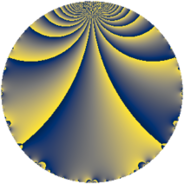# Properties

 Label 345.3.eLevel $345$ Weight $3$ Character orbit 345.e Rep. character $\chi_{345}(116,\cdot)$ Character field $\Q$ Dimension $60$ Newform subspaces $1$ Sturm bound $144$ Trace bound $0$

# Learn more about

## Defining parameters

 Level: $$N$$ $$=$$ $$345 = 3 \cdot 5 \cdot 23$$ Weight: $$k$$ $$=$$ $$3$$ Character orbit: $$[\chi]$$ $$=$$ 345.e (of order $$2$$ and degree $$1$$) Character conductor: $$\operatorname{cond}(\chi)$$ $$=$$ $$3$$ Character field: $$\Q$$ Newform subspaces: $$1$$ Sturm bound: $$144$$ Trace bound: $$0$$

## Dimensions

The following table gives the dimensions of various subspaces of $$M_{3}(345, [\chi])$$.

Total New Old
Modular forms 100 60 40
Cusp forms 92 60 32
Eisenstein series 8 0 8

## Trace form

 $$60q + 8q^{3} - 128q^{4} - 22q^{6} + 24q^{7} + 20q^{9} + O(q^{10})$$ $$60q + 8q^{3} - 128q^{4} - 22q^{6} + 24q^{7} + 20q^{9} - 38q^{12} - 64q^{13} + 20q^{15} + 288q^{16} + 90q^{18} - 24q^{19} - 28q^{21} + 120q^{22} - 36q^{24} - 300q^{25} - 88q^{27} - 248q^{28} + 80q^{30} + 152q^{31} - 64q^{33} - 24q^{34} - 126q^{36} + 76q^{39} + 176q^{42} - 32q^{43} + 326q^{48} + 524q^{49} - 20q^{51} + 188q^{52} - 436q^{54} - 112q^{57} - 180q^{58} - 20q^{60} - 224q^{61} + 384q^{63} - 116q^{64} - 564q^{66} - 520q^{67} - 120q^{70} + 60q^{72} + 464q^{73} - 40q^{75} + 176q^{76} + 422q^{78} - 24q^{79} + 316q^{81} - 148q^{82} + 264q^{84} - 120q^{85} - 452q^{87} - 392q^{88} + 180q^{90} + 568q^{91} + 292q^{93} - 364q^{94} - 378q^{96} + 464q^{97} - 488q^{99} + O(q^{100})$$

## Decomposition of $$S_{3}^{\mathrm{new}}(345, [\chi])$$ into newform subspaces

Label Dim. $$A$$ Field CM Traces $q$-expansion
$$a_2$$ $$a_3$$ $$a_5$$ $$a_7$$
345.3.e.a $$60$$ $$9.401$$ None $$0$$ $$8$$ $$0$$ $$24$$

## Decomposition of $$S_{3}^{\mathrm{old}}(345, [\chi])$$ into lower level spaces

$$S_{3}^{\mathrm{old}}(345, [\chi]) \cong$$ $$S_{3}^{\mathrm{new}}(15, [\chi])$$$$^{\oplus 2}$$$$\oplus$$$$S_{3}^{\mathrm{new}}(69, [\chi])$$$$^{\oplus 2}$$